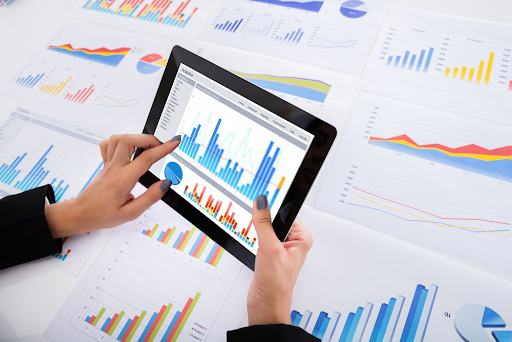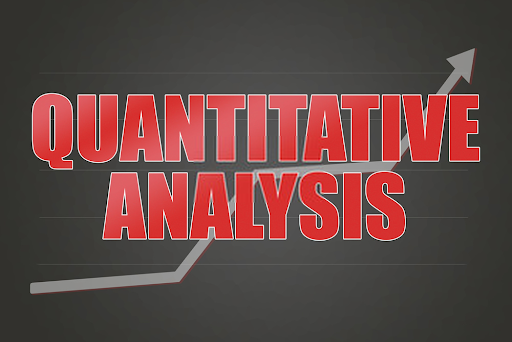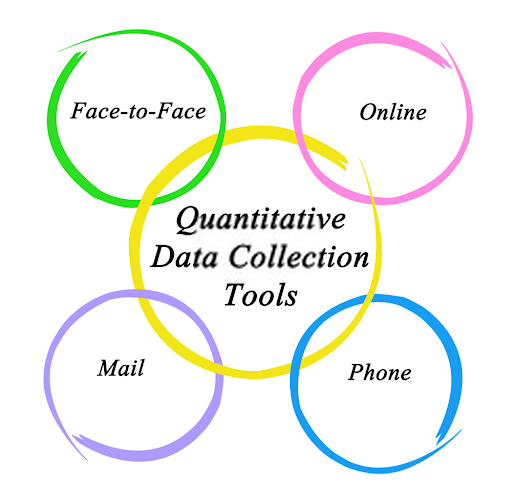Quantitative data is the type of data whose value is measured in the form of numbers or counts, with a unique numerical value associated with each data set. Also known as numerical data, quantitative data further describes numeric variables (e.g. How many? How often? How much?)

This data type can also be defined as a group of quantifiable information that can be used for mathematical computations and statistical analysis which informs real-life decisions. For example, a manufacturing company will need an answer to the question, “How much is the production cost?”.

A quantitative data of the company's cost of production will be collected through this question and will inform the company's selling cost (where selling cost = production cost + profit).

## Types of Quantitative Data

Quantitative Data can be divided into two types, namely; Discrete & Continuous Data.

• Discrete Data

Discrete data is a type of data that consists of counting numbers only, and as such cannot be measured. Measurements like weight, length, height are not classified under discrete data.

Examples of discrete data include; the number of students in a class, the number of days in a year, the age of an individual, etc. When trying to identify discrete data, we ask the following questions; Can it be counted? Can it be divided into smaller parts?

Discrete data can be said to be either countably finite or countably infinite. An example of a countably finite data is an arbitrary set A = {1, 2, 3,...,n; where n is less than infinity} while that of a countably infinite data is an arbitrary set B = {1,2,3,...}.

Also known as attribute data, discrete data can't be broken down into smaller units. It is typically counted in whole numbers and there is nothing like half a value.

• Continuous Data

Continuous data is a data type that takes on numeric values that can be meaningfully broken down into smaller units. As opposed to discrete data which can't be measured, continuous data can be placed on a measurement scale (e.g. weight, length, time, etc.).

Continuous data can be said to be either uncountably finite or uncountably infinite. For example, let us consider the Cumulative Grade Point (CGPA) of students in a class, measured on a 5 point scale.

A student can score any grade between 0 points and 5 points, including figures like 1.573, 4.5, 2.6981, etc. We classify this an uncountably finite continuous data because it has an upper (5) and lower bound (0).

An example of an uncountably infinite data is the set of real numbers, R = {..., -1, 0, 1, ...}. In this case, the data has neither an upper nor a lower bound.

Continuous data can also be divided into two types, namely; ratio data and interval data

Interval data is defined as type of data which is measured along a scale, in which each point is placed at equal distance from one another. It is an extension of ordinal data, with a standardised scale as opposed to the former.

Ratio data on the other hand, is an extension of interval data. It is the ultimate when we talk about data measurement because it tells us about the order, exact distance between units on the scale, and has an absolute zero.

The above characteristics of ratio data allows for the application of a wide range of inferential and descriptive statistical methods.

## What are Quantitative Data Examples?

There are various Quantitative data examples which are applied in both research and statistics. These examples vary and will therefore be separately highlighted below. ﻿﻿﻿﻿

### Quantitative Data Examples in Research

• Data projection

Researchers project future data using algorithms and mathematical analysis tools. For instance, a company who is about to launch a new product into the market will analyse quantitative data from previous research to predict an increase or decrease in sales.

• Census

The Government carry out census to acquire and record information about the members of a given population. Large government research departments uses census data to predict which sector of the economy needs money and how much they need, how many seats a state will have in the U.S. House of Representatives, etc.

• Annual income

When setting the selling price of a product, businesses use quantitative data of the annual income of a person or household to determine their purchasing power. This exercise is part of business research process and may be conducted before launching a new product or increasing the price of an existing product.

• Counter

Many online businesses use this to determine the number of website visits they get daily, number of product downloads on the app store, the number of users etc. The numbers are usually automatically generated through pre-programmed codes.

• Customer Satisfaction Survey

This is a case of quantification of qualitative entities used by businesses to improve their customer service. For example, telling a customer to rate an addition to a menu on a scale of 1-10 will help the restaurant decide whether to remove it, improve on it or leave it as it is.

### Quantitative Data Examples in Statistics

• Mean height in a class

The mean height of the students in a class will be calculated by recording the height of each student, adding it up and dividing it by the number of students in the class. A school might need to calculate the mean height of students in order to determine how high or low their chairs and tables should be.

• Measurement of physical objects

It may be used to record the length or width of an object. For example, when assigning a cubicle or office space to a new employee, HR may need to measure its length and breadth, then allocate according to their position or experience level.

• CGPA Calculation

The CGPA of a student is calculated by finding the average of the grade points of the student.

• Number generator

A computer program may be written to generate a particular set of numbers with a uniform (0,1) distribution. The accuracy of the number generator can be determined using the Chi-Squared Goodness of fit test. This will compare the counts generated with the expected count and determine whether they are accurate or not.

• Probability

The probability of an event occurring is calculated using the quantitative data of the ratio between the ways of achieving success and the total number of outcomes. The probability of a certain event is 1, impossible event is 0 and failure is 1 minus success.

## Characteristics of Quantitative Data

• Types

Quantitative data is of two types, namely; discrete and continuous data. Continuous data is further divided into interval and ratio data.

• Numerical Representation

Quantitative data takes up numeric values with numeric properties. Unlike categorical data which takes numeric values with descriptive characteristics, quantitative data exhibits numeric features.

• Order

There is a scale or order to quantitative data. For example, the numbers 1 to 3 can be written as 1,2,3 and 3,2,1 when arranged in ascending and descending orders respectively.

• Arithmetic Operation

One can perform arithmetic operations like addition and subtraction on quantitative data. Almost all statistical analysis methods can be carried out using quantitative data.

• Standardised Scale

Quantitative data has a standardised measurement scale. As opposed to ordinal data that has an order, but no standard scale.

• Analysis

Quantitative data can be analysed using descriptive and inferential statistical methods, depending on the aim of the research.

• Data Visualisation

Some of the data visualisation techniques adopted by quantitative data include; scatter plot, dot plot, stacked dot plot, histograms, etc.## Quantitative Data Analysis & Interpretation

### Data Preparation

The first stage of quantitative data analysis and interpretation is data preparation, where raw data is converted into something meaningful and readable. There are four steps of data preparation:

#### Step 1: Data Validation

This is done so as to find out whether the data collection was done without any bias. The process includes:

• Testing for fraud by checking whether all the respondents were truly interviewed or not.
• Screening the respondents to know whether they really met the research criteria.
• Checking whether the right procedure was followed.
• Checking whether the investigation is complete.

Researchers do this by picking a random sample from a large population.

#### Step 2: Data Editing

Large data sets may inevitably include errors, and that is why they need to be edited. During this process, data is inspected for completeness and consistency.

For example, a respondent may leave a field blank, which is a case of incompleteness. In another case, we may have a respondent who answered that he/she has no children also claim in another part that his(er) first child is in high school — this data is inconsistent.

#### Step 3: Coding and Data Entry

This is the process of quantifying qualitative data for easy analysis. It involves grouping and assigning values to survey responses. E.g. Male - 1, Female - 2.

#### Step 4: Data Transformation

This is the process of changing data into new format. For example, reducing a 5 point likert-type scale into 3 categories.

Consider a 5 point likert-type scale with the options very good, good, neutral, bad and very bad. This may be reduced into good, neutral and bad.### Quantitative Data Analysis Methods

After completing the first stage, the data is ready for analysis. There are two main data analysis methods used,  namely; descriptive statistics and inferential statistics.

#### Descriptive Statistical Method

Researchers make use of descriptive statistics to summarise quantitative data. It is often used when analysing a single variable, and as such is sometimes called univariate analysis. Some common descriptive statistical methods include:

• Mean: the average of a set of numbers.
• Median: the middle number of a set of numbers.
• Mode: most occuring number from a set of numbers.
• Range: the difference between the highest and lowest numbers from a set of numbers.

#### Inferential Statistical Method

This method measures the relationship (similarities and differences) between multiple variables to generate results and infer conclusions. Some examples of inferential statistics include; correlation, regression, ANOVA, etc.

There are also some other approaches to inferential statistics used to analyse real life data or surveys. They include:

• Cross-tabulation

Cross tabulation method uses basic tables to draw inferences between different data sets. The data-sets are matched and placed in the same row or column. Inferences are drawn by studying the similarities and differences between the data in each row or column as the case may be.

• MaxDiff analysis

MaxDiff analysis method is used to gauge respondent’s preference for a particular set of options. It determines the most prefered and the least preferred. This data is compared for various samples to infer a conclusion.

• TURF analysis

Turf Analysis is mostly used by businesses to determine their go to market strategy. For example, a company may run the same ad across different social media platforms and analyse the number of customers reached for each platform to determine the best place to run ads.

• Gap analysis

Gap analysis measures gaps in performance and how to breach this gap. It uses a side-by-side matrix to measure the difference between these performances.

• Text analysis

Text analysis is used to extract useful information from a text document. Computers use this method to disintegrate unstructured text document and convert them into structured data.

• It allows for a broader study, using different statistical methods. This is mainly because quantitative data is compatible with most statistical analysis methods.
• It summarises data and streamlines it into relevant information. Quantitative data is numeric in nature, and as such codes qualitative data into numbers before analysis. This is sometimes called quantification of qualitative data.
• Research replication is made easy with quantitative data. Therefore making comparisons across categories and over time. This is due to the fact that quantitative research uses a standardised procedure.
• By coding qualitative data into unknown numbers, researchers are able to eliminate personal bias that may arise from handling information of subjects known to them.

• They collect a narrow data set and may end up losing out on some relevant information.
• The results do not provide elaborate account of human feelings and perceptions.
• Research is carried out using standardised rules which gives results that may not be applicable in the real world scenario.
• It uses preset answers which does not capture enough details and human emotions.
• Preset answers is a reflection of the researcher's point of view rather than that of the respondent.## What is the best data collection tool for quantitative data?

Formplus is the best tool for collecting quantitative data. It has different features for building online surveys for collecting quantitative data and analysis automation for the data collected.

This web based form builder helps businesses create better campaigns, choose the best marketing channels to increase their sales and improve customer satisfaction. As a researcher, you no longer have to share printed forms to respondents and manually input the data for analysis.

Formplus eliminates stress and need for manpower by providing an all in one form builder with a sharing option that makes it easy for you to share the forms to respondents. The data collection process is also automated, while eliminating human error.

## How to Use Formplus Builder to Collect Quantitative Data ( Step-by-step-guide)

To collect quantitative data using formplus builder, follow these steps:

• Visit www.formpl.us on your desktop or mobile device.### Step 2: Start Creating Forms

Formplus gives you a 21-day free trial to test all features and start collecting quantitative data from online surveys. Pricing plan starts after trial expiration at \$20 monthly, with reasonable discounts for Education and Non-Governmental Organizations.

• Click on the Create form button to start creating forms for free.
• You can also click on the Upgrade Now button to upgrade to a pricing plan at \$20 monthly.### Step 3: Collect Quantitative Data

We will be creating a sample quantitative data collection form that inputs student's scores (First test score and final test score), then output the total grade in percentage.

• Edit form title and click on the input section of the form builder menu.
• The input sections lets you insert features such as small texts for names, numbers, date, email, long text for general feedback. Click on the Number tab to input numbers.• You can customise the input fields in the settings section. Click save when done.• Click on the Advanced inputs section of the form builder menu.• The Add Calculation menu lets you perform arithmetic operations on the input.• Use the formula;

(First test score+Final test score) × 100 / 200 = (First test score+Final test score)/2 to calculate the Total Grade in percentage.• Click on continue to get back to the form builder section.You can also customise your forms, share to respondents and view response analytics.

## Conclusion

Quantitative data is perhaps the most widely used data type in research. This is partly due to its ease of computation and compatibility with most statistical analysis methods.

In quantitative data analysis, we take a sample population, classify its features, and even construct more complex statistical models in an attempt to explain what is observed. Our findings may be extended to a larger population, and comparisons may be made between two sample populations.

Quantitative data is collected through a standard procedure, making it easy for researchers to replicate past research or build on current ones.

• Formplus Blog

You may also like:

###### Primary Data: Definition, Examples & Collection Techniques

One of the major elements and basis of statistical research is data collection, where the most basic data that can be collected in this ...

Formplus Blog
###### What is Numerical Data? [Examples,Variables & Analysis]

When working with statistical data, researchers need to get acquainted with the data types used—categorical and numerical data. The ...

Formplus Blog
###### Qualitative Data: Collection Techniques, Examples & Analysis

Qualitative data collection process may be assessed through two different points of view—that of the questionnaire and the respondents. A ...

Formplus Blog